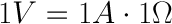top of page
Search

# What's In A Name?

There are three basic principles to electricity, hence Principles Electric. When it comes to electricity these three basic building blocks are voltage, current, and resistance. These three pieces are required to manipulate and utilize electricity. Simply put, electricity is the flow of electric current along a conductor. This current takes the form of free electrons that transfer from one atom to the next. The more electrons, the better it conducts. Sometimes these three are called the volt, the ampere, and the ohm.This brings is to the next part, Ohm’s law! Georg Ohm was a Bavarian scientist who studied electricity. First by describing a unit of resistance that is defined by current and voltage.

1. The Volt

The pressure that is put on free electrons that causes them to flow is known as electromotive force (EMF). The volt is the unit of pressure, i.e., the volt is the amount of electromotive force required to push a current of one ampere through a conductor with a resistance of one ohm.

2. The Ampere

The ampere defines the flow rate of electric current. For instance, when one coulomb (or 6 x 1018 electrons) flows past a given point on a conductor in one second, it is defined as a current of one ampere.

3. The OhmThe ohm is the unit of resistance in a conductor. Three things determine the amount of resistance in a conductor: its size, its material, e.g., copper or aluminum, and its temperature.

A conductor’s resistance increases as its length increases or diameter decreases. The more conductive the materials used, the lower the conductor resistance becomes. Conversely, a rise in temperature will generally increase resistance in a conductor.

Ohm’s Law defines the correlation between electric current (I), voltage (V), and resistance (R) in a conductor.

Where: V = volts, I = amps, R = ohms

Let's say, for example, that we have a circuit with the potential of 1 volt, a current of 1 amp, and resistance of 1 ohm. Using Ohm's Law we can say:To learn more about Ohm’s law, the Principles of Electricity, or for a fun summer science experiment with the kids check out the amazing site we reference in this blog: SparkFun